# Class 12 Maths NCERT Solutions for Chapter 6 Application of Derivatives Exercise 6.3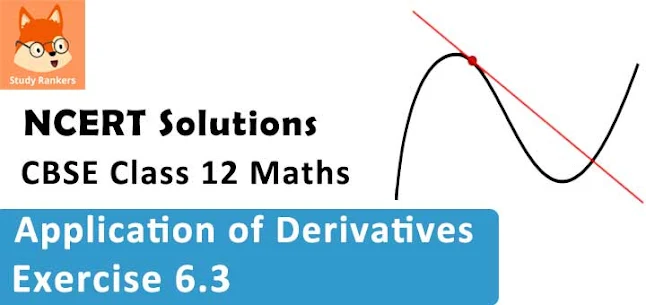### Application of Derivatives Exercise 6.3 Solutions

1. Find the slope of the tangent to the curve y = 3x4 − 4x at x = 4.

Solution

The given curve is y = 3x4 − 4x.
Then, the slope of the tangent to the given curve at x = 4 is given by,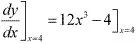= 12(4)3 - 4 = 12(64) - 4 = 764

2. Find the slope of the tangent to the curve y = (x -1)/(x - 2), x != 2 at x = 10.

Solution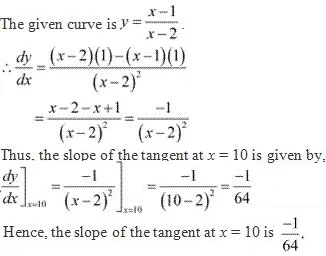3. Find the slope of the tangent to curve y = x3 − + 1 at the point whose x-coordinate is 2.
Solution
The given curve is y = x3 - x + 1.
∴ dy/dx = 3x2 - 1.
The slope of the tangent to a curve a (x0 , y0) is dy/dx .
It is given that x0 = 2.
Hence, the slope of the tangent at the point where the x - coordinate is 2 is given by,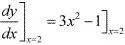= 3(2)2 - 1 = 12 - 1 = 11

4. Find the slope of the tangent to the curve y = x3 − 3x + 2 at the point whose x-coordinate is 3.
Solution
The given curve is y = x3 − 3x + 2 .
∴ dy/dx = 3x2 - 3
The slope of the tangent to a curve at  (x0 , y0) is dy/dx](x0 , y0) .
Hence, the slope of the tangent at the point where the x - coordinate is 3 is given by,
dy/dx]x-3 = 3x2 - 3]x-3 = 3(3)2 - 3 = 2 -3 = 24

5. Find the slope of the normal to the curve x = acos3θ, y = asin3θ at θ = π/4 .
Solution
It is given that x = acos3θ, and y = asin3θ .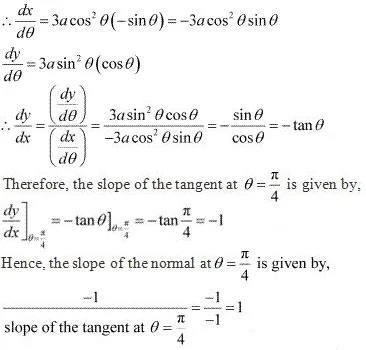6. Find the slope of the normal to the curve x = 1 - a sin θ and y = b cos2 θ at θ = π/2.
Solution
It is given that x = 1 - a sin θ and y = b cos2 θ .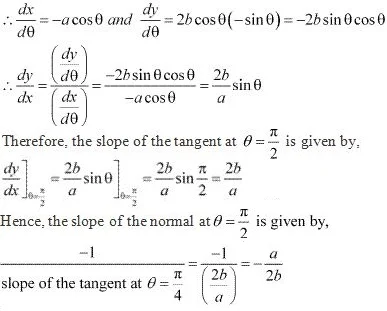7. Find points at which the tangent to the curve y = x3 − 3x2 − 9x + 7 is parallel to the x-axis.
Solution
The equation of the given curve is y = x3 − 3x2 − 9x + 7 .
∴ dy/dx = 3x2 - 6x - 9
Now, the tangent is parallel to the x- axis if the slope of the tangent is zero.
∴ 3x2 - 6x - 9 = 0
⇒ x2 - 2x - 3 = 0
⇒ (x -3)(x + 1) = 0
⇒ x = 3 or x = -1
When x = 3, y = (3)3 - 3(3)2 - 9(3) + 7 = 27 - 27 - 27 + 7 = -20.
When x = -1, y = (-1)3 - 3(-1)2 - 9(-1) + 7 = -1 - 3 + 9 + 7 = 12.
Hence, the points at which the tangent is parallel to the x - axis are (3, -20) and (-1, 12).

8. Find a point on the curve y = (x − 2)2 at which the tangent is parallel to the chord joining the points (2, 0) and (4, 4).
Solution
If a tangent is parallel to the chord joining the points (2, 0) and (4, 4), then the slope of the tangent = the slope of the chord.
The slope of the chord is (4- 0)/(4 - 2) = 4/2 = 2.
Now, the slope of the tangent to the given curve at a point (x, y) is given by,
dy/dx = 2(x - 2)
Since the slope of the tangent = slope of the chord, we have :
2(x - 2) = 2
⇒ x - 2 = 1
⇒ x = 3
When x = 3, y = (3 - 2)2 = 1.
Hence, the required point is (3, 1).

9. Find the point on the curve y = x3 − 11x + 5 at which the tangent is y = x − 11.
Solution
The equation of the given curve is y =  x3 − 11x + 5 .
The equation of the tangent to the given curve is given as y = x - 11 (which is of the form y =  mx + c)
∴ Slope of the tangent = 1
Now, the slope of the tangent to the given curve at the point (x, y) is given by, dy/dx = 3x2 - 11
Then, we have :
3x2 - 11 = 1
⇒ 3x2 = 12
⇒ x2 = 4
⇒ x = ± 2
When x = 2, y = (2)3 - 11(2) + 5 = 8 - 22 + 5 = -9.
When x = -2, y = (-2)3 - 11(-2) +5 = -8 + 22 + 5 = 19.
Hence, the required points are (2, -9) and (-2, 19).

10. Find the equation of all lines having slope −1 that are tangents to the curve y = 1/(x-1), x ≠ 1 .
Solution
The equation of the given curve is y = 1/(x-1), x ≠ 1 .
The slope of the tangents to the given curve at any point (x, y) is given by,
dy/dx = -1/(x - 1)2
If the slope of the tangent is -1, then we have :
-1/(x - 1)2 = -1
⇒ (x - 1)2 = 1
⇒ x - 1 = ± 1
⇒ x = 2, 0
When x = 0, y = -1 and when x = 2, y = 1.
Thus, there are two tangents to the given curve having slope -1. These are passing through the points (0, -1) and (2, 1).
∴ The equation of the tangent through (0, -1) is given by,
y - (-1) = -1(x - 0)
⇒ y + 1 = -x
⇒ y + x + 1 = 0
∴ The equation of  the tangent through (2, 1) is given by,
y - 1 = -1(x - 2)
⇒ y - 1 = -x + 2
⇒ y + x - 3 = 0
Hence, the equations of the required lines are y + x + 1 = 0 and y + x - 3 = 0.

11. Find the equation of all lines having slope 2 which are tangents to the curve y = 1/(x - 3), x ≠ 3.
Solution
The equation of the given curve is y = 1/(x - 3), x ≠ 3.
The slope of the tangent to the given curve at any point (x, y)  is given by,
dy/dx = -1/(x - 3)2
If the slope of the tangent is 2, then we have :
-1/(x- 3)2 = 2
⇒ 2(x - 3)2 = -1
⇒ (x - 3)2 = -1/2
This is not possible since the L.H.S. is positive while the R.H.S. is negative .
Hence, there is no tangent to the given curve having slope 2.

12. Find the equations of all lines having slope 0 which are tangent to the curve y = 1/(x2 - 2x + 3) .
Solution
The slope of the tangent to the given curve at any point (x, y) is given by,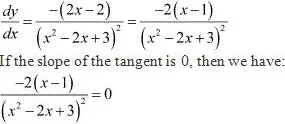⇒ - 2(x - 1) = 0
⇒ x = 1
When x = 1, y = 1/(1 - 2 + 3) = 1/2.
∴ The equation of the tangent through (1, 1/2) is given by,
y - 1/2 = 0 (x - 1)
⇒ y - 1/2 = 0
⇒ y = 1/2
Hence, the equation of the required line is y = 1/2.

13. Find points on the curve x2/9 + y2/16 =1 at which the tangent are
(i) parallel to x - axis
(ii) parallel to y - axis
Solution
The equation of the given curve is x2/9 + y2/16 =1 .
On differentiating both sides with respect to x, we have :
2x/9 + 2y/16 .dy/dx = 0
⇒ dy/dx = -16x/9y

(i) The tangent is parallel to the x - axis if the slope of the tangent is 0 i.e., -16x/9y = 0, which is possible if x = 0.
Then, x2/9 + y2/16 =1 for x = 0
⇒ y2 = 16
⇒ y = ± 4
Hence, the points at which the tangents are parallel to the x - axis are (0, 4) and (0, -4).

(ii) The tangent is parallel to the y - axis if the slope of the normal is 0, which gives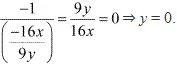Then, x2 /9 + y2 /16 = 1 for y = 0.
⇒ x = ± 3
Hence, the points at which the tangents are parallel to the y - axis are (3, 0) and (-3, 0).

14. Find the equations of the tangent and normal to the given curves at the indicated points:
(i) y = x4 − 6x3 + 13x2 − 10x + 5 at (0, 5)
(ii) y = x4 − 6x3 + 13x2 − 10x + 5 at (1, 3)
(iii) y = x3 at (1, 1)
(iv) x2 at (0, 0)
(v) x = cos t, y = sin t at  t = π/4
Solution
(i) The equation of the curve is y = x4 − 6x3 + 13x2 − 10x + 5
On differentiating with respect to x, we get :
dy/dx = 4x3 - 18x2 + 26x - 10
dy/dx](0, 5) = -10
Thus, the slope of the tangent at (0, 5) is -10. The equation of the tangent is given as :
y - 5 = -10(x - 0)
⇒ y - 5 = -10x
⇒ 10x + y = 5
The slope of the normal at (0, 5) is -1/[Slope of the tangent at (0, 5)] = 1/10 .
Therefore, the equation of the normal at (0, 5) is given as :
y - 5 = 1/10 (x - 0)
⇒ 10y - 50  = x
⇒ x - 10y + 50 = 0

(ii) The equation of the curve is y = x4 - 6x3 + 13x2 - 10x + 5.
On differentiating with respect to x, we get :
dy/dx = 4x3 - 18x2 + 26x - 10
dy/dx](1, 3) = 4 - 18 + 26 - 10 = 2
Thus, the slope of the tangent at (1, 3) is 2. The equation of the tangent is given as :
y - 3 = 2(x - 1)
⇒ y - 3 = 2x - 2
⇒ y = 2x + 1
The slope of the normal at (1, 3) is -1/[Slope of the tangent at (1, 3)] = -1/2 .
Therefore, the equation of the normal at (1, 3) is given as :
y - 3 = -1/2 (x - 1)
⇒ 2y - 6 = -x + 1
⇒ x + 2y - 7 = 0

(iii) The equation of the curve is y = x3 .
On differentiating with respect to x, we get :
dy/dx = 3x2
dy/dx](1, 1) = 3(1)2 = 3
Thus, the slope of the tangent at (1, 1) is 3 and the equation of the tangent is given as :
y - 1 = 3(x - 1)
⇒ y = 3x - 2
The slope of the normal at (1, 1) is -1/[Slope of the tangent at (1, 1)] = -1/3 .
Therefore, the equation of the normal at (1, 1) is given as :
y - 1 = -1/3 (x - 1)
⇒ 3y - 3 = - x + 1
⇒ x + 3y - 4 = 0

(iv) The equation of the curve is y = x2 .
On differentiating with respect to x, we get :
dy/dx = 2x
dy/dx](0, 0) = 0
Thus, the slope of the tangent at (0, 0) is 0 and the equation of the tangent is given as :
y - 0 = 0(x - 0)
⇒ y = 0
The slope of the normal at (0, 0) is -1/[Slope of the tangent at (0, 0)] = -1/0 , which is not defined.
Therefore, the equation of the normal at (x0 , y0) = (0, 0) is given by
x = x0 = 0.

(v) The equation of the curve is x = cos t, y = sin t.
x = cos t and y = sin t
∴ dx/dt = - sin t, dy/dt = cos t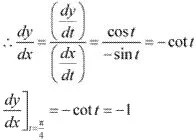∴ The slope of the tangent at t = π/4 is -1.
When t = π/4 , x = 1/√2 and y = 1/√2 .
Thus, the equation of the tangent to the given curve at t = π/4 i.e., at  [(1/√2 , 1/√2)] is
y - 1/√2 = -1 (x - 1/√2) .
⇒ x + y - 1/√2 - 1/√2 = 0
⇒ x + y - √2 = 0
The slope of the normal at t = π/4 is -1/[Slope of the tangent at t = π/4] = 1.
Therefore, the equation of the normal to the given curve at t = π/4 i.e., at [(1/√2 , 1/√2)] is
y - 1/√2 = 1 (x - 1/√2)
⇒ x = y

15. Find the equation of the tangent line to the curve y = x2 − 2x + 7 which is
(a) parallel to the line 2x − y + 9 = 0.
(b) perpendicular to the line 5y - 15x = 13
Solution
The equation of the given curve is y =  x2 − 2x + 7 .
On differentiating with respect to x, we get :
dy/dx = 2x - 2

(a) The equation of the line is 2x - y + 9 = 0.
2x - y + 9 = 0 ⇒ y = 2x + 9
This is of the form y = mx + c .
∴ Slope of the line = 2
If a tangent is parallel to the line 2x - y + 9 = 0, then the slope of the tangent is equal to the slope of the line.
Therefore, we have :
2 = 2x - 2
⇒ 2x = 4
⇒ x = 2
Now, x = 2
⇒ y = 4 - 4 + 7 = 7
Thus, the equation of the tangent passing through (2, 7) is given by,
y - 7 = 2(x - 2)
⇒ y- 2x - 3 = 0
Hence, the equation of the tangent line to the given curve (which is parallel to line 2x - y + 9 = 0 ) is y - 2x - 3 = 0.

(b) The equation of the line is 5y - 15x = 13.
5y - 15x = 13 ⇒ y = 3x + 13/5
This is of the form y = 2x + c .
∴ Slope of the line  = 3
If a tangent is perpendicular to the line 5y - 15x = 13, then the slope of the tangent is -1/[slope of the line] = -1/3 .
⇒ 2x - 2 = -1/3
⇒ 2x = -1/3 + 2
⇒ 2x = 5/3
⇒ x = 5/6
Now, x = 5/6
⇒ y = (25/36) -(10/6) + 7 = (25 - 60 + 252)/36 = 217/36
Thus, the equation of the tangent passing through (5/6, 217/36) is given by,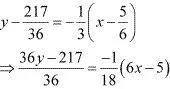⇒ 36y - 217 = -2(6x - 5)
⇒ 36y - 217 = -12x + 10
⇒ 36y + 12x - 227 = 0
Hence, the equation of the tangent line to the given curve (which is perpendicular to line 5y - 15x = 13) is 36y + 12x - 227 = 0.

16. Show that the tangents to the curve y = 7x3 + 11 at the points where x = 2 and x = −2 are parallel.
Solution
The equation of the given curve is y = 7x3 + 11.
∴ dy/dx = 21x2
The slope of the tangent to a curve at (x0 , y0) is dy/dx](x0 ,y0)
Therefore, the slope of the tangent at the point where x = 2 is given by,
dy/dx]x = 2 = 21(2)2 = 84
And, the slope of the tangent at the point where x = -2 is given by,
dy/dx]x = -2 = 21(2)2 = 84
It is observed that the slopes of the tangents at the points where x = 2 and x = -2 are equal.
Hence, the two tangents are parallel.

17. Find the points on the curve y = x3 at which the slope of the tangent is equal to the y-coordinate of the point.
Solution
The equation of the given curve is y = x3
∴ dy/dx = 3x2
The slope of the tangent at the point (x, y) is given by,
dy/dx](x, y) = 3x2
When the slope of the tangent is equal to the y - coordinate of the point, then y = 3x2
Also, we have y = x3 .
∴ 3x2 = x3
⇒ x2 (x - 3) = 0
⇒ x = 0, x = 3
When x = 0, they y = 0 and when x = 3, then y = 3 (3)2 = 27.
Hence, the required points are (0, 0) and (3, 27).

18. For the curve y = 4x3 − 2x5, find all the points at which the tangents passes through the origin.
Solution
The equation of the given curve is y = 4x3 − 2x5  .
∴ dy/dx = 12x2 - 10x4
Therefore, the slope of the tangent at a point (x, y) is 12x2 - 10x4 .
The equation of the tangent at (x, y) is given by,
Y - y = (12x2 - 10x4 )(X - x) ...(1)
When the tangent passes through the origin (0, 0), then X = Y = 0.
Therefore, equation (1) reduces to :
-y = (12x2 - 10x4 )(-x)
y = 12x3 - 10x5
Also, we have y = 4x3 - 2x5 ,
∴ 12x3 - 10x5 = 4x3 - 2x5
⇒ 8x5 - 8x3 = 0
⇒ x5 - x3 = 0
⇒ x3 (x2 - 1) = 0
⇒ x = 0, ± 1
When x = 0, y = 4(0)3 - 2(0)5 = 0.
When x = 1, y = 4(1)3 - 2(1)5 = 2.
When x = -1, y = 4(-1)3 - 2(-1)5 = -2.
Hence, the required points are (0, 0), (1, 2) and  (-1, -2).

19. Find the points on the curve x2 + y2 − 2x − 3 = 0 at which the tangents are parallel to the x-axis.
Solution
The equation of the given curve is x2 + y2 − 2x − 3 = 0 .
On differentiating with respect to x, we have :
2x + 2y(dy/dx) - 2 = 0
⇒ y. (dy/dx) = 1 - x
⇒ dy/dx = (1 - x)/y
Now, the tangents are parallel to the x - axis if the slope of the tangent is 0.
∴ (1 - x)/y = 0
⇒ 1 - x = 0
⇒ x = 1
But, x2 + y2 -2x - 3 = 0 for x = 1.
⇒ y2 = 4
⇒ y = ± 2
Hence, the points at which the tangents are parallel to the x - axis are (1, 2) and (1, -2).

20. Find the equation of the normal at the point (am2, am3) for the curve ay2 = x3.
Solution
The equation of the given curve is ay2 = x3 .
On differentiating with respect to x, we have :
2ay . (dy/dx) = 3x2
⇒ dy/dx = 3x2 /2ay
The slope of a tangent to the curve at (x0, y0) is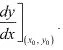⇒ The slope of the tangent to the given curve at (am2, am3) is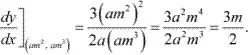∴ Slope of normal at (am2, am3)
= -1/[slope of the tangent at (am2, am3) = -2/3m
Hence, the equation of the normal at (am2, am3) is given by,
y - am3 = -2/3m (x - am2
⇒ 3my - 3am4 = -2x + 2am2
⇒ 2x + 3my - am2 (2 + 3m2) = 0

21. Find the equation of the normal to the curve y = x3 + 2+ 6 which are parallel to the line x + 14y + 4 = 0.
Solution
The equation of the given curve is y = x3 + 2x + 6.
The slope of the tangent to the given curve at any point (x, y) is given by,
dy/dx = 3x2 + 2
∴ Slope of the normal to the given curve at any point (x, y)
= -1/[Slope of the tangent at the point (x, y)]
= -1/(3x2 + 2)
The equation of the given line is x + 14y + 4 = 0.
x + 14y + 4 ⇒ y = -(1/14)x - 4/14 (which is of the form y = mx + c)
∴ Slope of the given line = -1/14
If the normal is parallel to the line, then we must have the slope of the normal being equal to the slope of the line.
∴ -1/(3x2 +2)   = -1/14
⇒ 3x3 + 2 = 14
⇒ 3x2 = 12
⇒ x2 = 4
⇒ x = ± 2
When x = 2, y = 8 + 4 + 6 = 18.
When x = -2, y = -8 - 4 + 6 = -6.
Therefore, there are two normals to the given curve with slope -1/14 and passing through the points (2, 18) and (-2, -6).
thus, the equation of the normal through (2, 18) is given by,
y - 18 = (-1/14)(x - 2)
⇒ 14y - 252 = -x + 2
⇒ x + 14y - 254 = 0
And, the equation of the normal through (-2, -6) is given by,
y -(-6) = -1/14 (x - 2)
⇒ 14y - 252 = -x + 2
⇒ x + 14y - 254 = 0
And, the equation of the normal through (-2, -6) is given by,
y - (-6)  = (-1/14) [x-(-2)]
⇒ y + 6 = (-1/14) (x + 2)
⇒ 14y + 84 = -x - 2
⇒ x + 14y + 86 = 0
Hence, the equations of the normals to the given curve (which are parallel to the given line ) are x + 14y - 254 = 0 and x + 14y + 86 = 0.

22. Find the equations of the tangent and normal to the parabola y2 = 4ax at the point (at2, 2at).
Solution
The equation of the given parabola is y2 = 4ax.
On differentiating y2 = 4ax with respect to x, we have:
2y . (dy/dx) = 4a
⇒ dy/dx = 2a/y
∴ The slope of the tangent at (at2 , 2at) is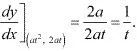Then, the equation of the tangent at (at2 , 2at) is given by,
y - 2at = 1/t (x - at2 )
⇒ ty - 2at2 = x - at2
⇒ ty = x + at2
Now, the slope of the normal at (at2 , 2at) is given by,
-1/[Slope of the tangent at (at2 , 2at)] = -t
Thus, the equation of the normal at (at2 , 2at) is given as :
y - 2at = -t(x - at2 )
⇒ y - 2at = -tx + at3
⇒ y = -tx + 2at + at3

23. Prove that the curves x = y2 and xy = k cut at right angles if 8k2 = 1. [Hint: Two curves intersect at right angle if the tangents to the curves at the point of intersection are perpendicular to each other.]
Solution
The equations of the given curves are given as x = y2 and xy = k.
Putting x = y2 in xy  = k, we get :
y3 = k
⇒ y = k1/3
∴ x = k2/3
Thus, the point of intersection of the given curves is (k2/3 , k1/3 ).
Differentiating x = y2 with respect to x, we have :
1 = 2y. (dy/dx)
⇒ dy/dx = 1/2y
Therefore, the slope of the tangent to the curve x = y2 at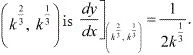On differentiating xy = k with respect to x, we have:
x. (dy/dx) + y = 0
⇒ dy/dx = -y/x
∴ Slope of the tangent to the curve xy = k at (k2/3, k1/3) is given by,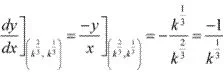We know that two curves intersect at right angles if the tangents to the curves at the point of intersection i.e., at (k2/3 , k1/3 ) are perpendicular to each other.
This implies that we should have the product of the tangents as -1.
Thus, the given two curves cut at right angles if the product of the slopes of their respective tangents at (k2/3 , k1/3) is -1.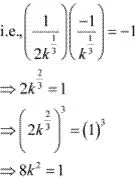Hence, the given two curves cut at right angels if 8k2 = 1.

24. Find the equations of the tangent and normal to the hyperbola x2/a2 - y2/b2 at the point (x0, y0).
Solution
Differentiating x2 /a2 - y2 /b2  = 1 with respect to x, we have :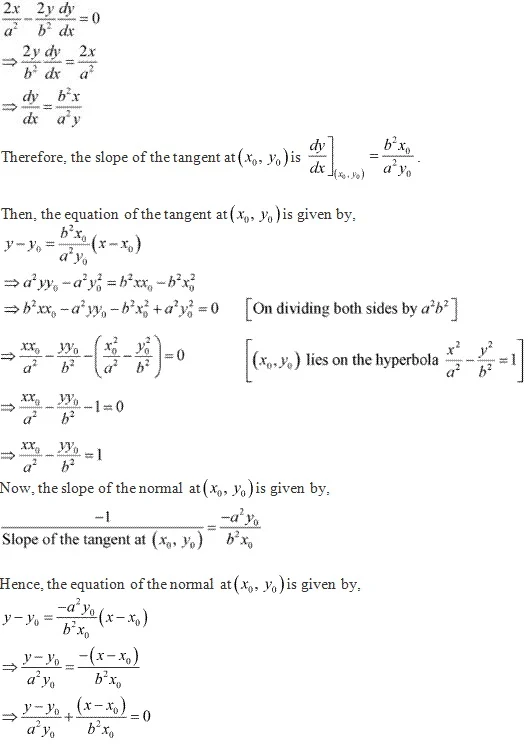25. Find the equation of the tangent to the curve y = √(3x-2) which is parallel to the line 4x − 2y + 5 = 0.
Solution
The equation of the given curve is y = √(3x-2) .
The slope of the tangent to the given curve at any point (x, y) is given by,
dy/dx = 3/2√(3x - 2)
The equation of the given line is 4x - 2y + 5 = 0.
4x - 2y + 5 = 0 ⇒ y = 2x + 5/2 which is of the form y = mx + c)
∴ Slope of the line = 2
Now, the tangent to the given curve is parallel to the line 4x -2y - 5 = 0 if the slope of  the tangent is equal to the slope of the line.
3/[2√(3x - 2)] = 2
⇒ √(3x - 2) = 3/4
⇒ 3x - 2 = 9/16
⇒ 3x = 9/16 + 2 = 41/16
⇒ x = 41/48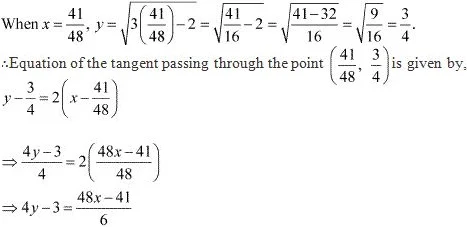⇒ 24y - 18 = 48x - 41
⇒ 48x - 24y = 23
Hence, the equation of the required tangent is  48x - 24y = 23

26. The slope of the normal to the curve y = 2x2 + 3 sin x at x = 0 is
(A) 3
(B) 1/3
(C) −3
(D) -1/3
Solution
The equation of the given curve is y = 2x2 + 3sin x.
Slope of the tangent to the given curve at x = 0 is given by,
dy/dx]x = 0 = 4x + 3 cos x]x = 0 = 0 + 3 cos 0 = 3
Hence, the slope of the normal to the given curve at x = 0 is
-1/[Slope of the tangent at x = 0] = -1/3 .

27. The line y = x + 1 is a tangent to the curve y2 = 4x at the point
(A) (1, 2)
(B) (2, 1)
(C) (1, −2)
(D) (−1, 2)
Solution
The equation of the given curve is  y2 = 4x .
Differentiating with respect to x, we have :
2y. dy/dx = 4 ⇒ dy/dx = 2/y
Therefore, the slope of the tangent to the given curve at any point (x, y) is given by,
dy/dx = 2/y
Therefore, the slope of the tangent to the given curve at any point (x, y) is given by,
dy/dx =2/y
The given line is y = x + 1 (which is of the form y = mx + c)
∴ Slope of the line = 1
The line y = x + 1 is a tangent to the given curve if the slope of the line is equal to the slope of the tangent. Also, the line must intersect the curve.
Thus, we must have :
2/y = 1
⇒ y = 2
Now,
y = x + 1
⇒ x = y- 1
⇒ x = 2 - 1 = 1
Hence, the line y = x + 1 is a tangent to the given curve at the point (1, 2).